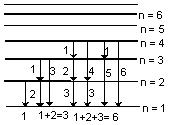Click to Chat

1800-1023-196

+91-120-4616500

CART 0

• 0

MY CART (5)

Use Coupon: CART20 and get 20% off on all online Study Material

ITEM
DETAILS
MRP
DISCOUNT
FINAL PRICE
Total Price: Rs.

There are no items in this cart.
Continue Shopping```Solved Questions of Atomic StructureProblem 1: Why Bohr’s orbits are called stationary states?

Solution: This is because the energies of orbits in which the electrons revolve are fixed.

Problem 2: Explain why the electronic configuration of Cu is 3d104s1 and not 3d94s2.

Solution: In the 3d104s1 the d-sub shell is completely filled which is more stable.

Problem 3: Fe3+ ion is more stable than Fe2+ ion. Why?

Solution: In Fe3+ ion 3d sub shell is half filled hence more stable configuration.

Problem 4: Calculate the accelerating potential that must be applied to a proton beam to give it an effective wavelength of 0.005 nm.

Solution:

v = h/mλ

ev = 1/2mv2

Putting the values we get

V = 32.85 volt

Problem 5: Give one example of isodiapheres.

Solution: Isodiapheres have same difference between the number of neutrons and protons. For example

3919K & 3115P

n-p=1     m-p=1

Problem 6: Which electronic transition in Balmer series of hydrogen atom has same frequency as that of n = 6 to n = 4 transition in He+. [Neglect reduced mass effect].

Solution :

v-He+ = RZ2 [ 1/42 - 1/62]

= 4R [ 36 - 16/36 x 16 ] = 5R/36

v-H = R x 12 [ 1/22 - 1/n2]

∴ v-He+ = v-H

5R/36 = R/4 - R/n2

On solving above equation

n2 = 9

∴n = 3

Or corresponding transition from 3 → 2 in Balmer series of hydrogen atom has same frequency as that of 6 → 4 transition in He+.

Problem 7: Calculate ionization potential in volts of (a) He+ and (b) Li2+

Solution:

I.E. = 13.6Z2/n2

=  13.6 x Z2 \12 [Z =2 for He+]

= 13.6 x 4 = 54.4 eV

Similarly for Li2+ = 13.6 x 32/12

= 13.6 x 9 = 122.4 eV

Problem 8: Calculate the ratio of K.E and P.E of an electron in an orbit?

Solution:

K.E. = Ze2/2r

P.E. = -Ze2/r

∴ P.E. = –2K.E

∴ K.E/P.E = - 1/2

Problem 9: How many spectral lines are emitted by atomic hydrogen excited to  nth energy level?

Solution:Thus the number of lines emitted from nth energy level

= 1 + 2 + 3 +………… n – 1 = ∑(n – 1)

∑n =  n(n+1)/2

∴ ∑ (n – 1) = ( n-1) (n-1+1)/2  = (n-1) (n)/2

Number of spectral lines that appear in hydrogen spectrum when an electron jumps from nth energy level = n (n-1)/2

Problem 10:  Calculate (a) the de Broglie wavelength of an electron moving with a velocity of 5.0x 105 ms–1 and (b) relative de Broglie wavelength of an atom of hydrogen and atom of oxygen moving with the same velocity (h = 6.63 x 10–34 kg m2 s–1)

Solution:

(a  λ = h/mv  = 6.63 x 10-34 kgm2s-2/ (9.11 x 10-31kg) ( 5.0 x 105ms-1)

Wavelength λ = 1.46 x10–9m

(b) An atom of oxygen has approximately 16 times the mass of an atom of hydrogen. In the formula λ = h/mv, h is constant while the conditions of problem make v, also constant. This means that λ and m are variables and λ varies inversely with m. Therefore, λ for the hydrogen atom would be 16 times greater than λ for oxygen atom.

Problem 11: A 1 MeV proton is sent against a gold leaf (Z = 79). Calculate the distance of closest approach for head on collision.

Solution: K.E. = P.E.

1/2mv2 = 1/4πεo  Ze2/d

Hence d =  Ze2/4πεo( 1/2mv2)

= 9 x 109 x 79 x ( 1.6 x 10-19)2 / ( 1.6 x 10-13 )   [ as 1 MeV = 1.6 x 10-13 J]

= 1.137 x 10-13 m

Problem 12: What is the wavelength associated with 150 eV electron

Solution:

λ = h/√2 x m x K.E.

= 6.626 x 1-34Js / √ 2 x 9.1 x 10-31kg x 150 x 1.6 x 10-19 J   = 6.626 x 10-34 / √4368 x 10-50 = 10–10 m = 1 Å

Problem 13: The energy of electron in the second and third Bohr orbit of the hydrogen atom is –5.42 x  10–12 erg and –2.41 x  10–12erg, respectively. Calculate the wavelengths of emitted radiation when the electron drops from third to second orbit.

Solution: E3 – E2 = hv = hc/λ – 2.41 ´ 10–12 – (– 5.42 ´ 10–12) = 6.626 x 10-27 x 3 x 1010/ λ

∴ λ = 6.626 x 10-27 x 3 x 1010 / 3.01 x 10-12

= 6.604 x 10–5 cm = 6.604 x 10–5 x 108 = 6604 Å

Problem 14: O2 undergoes photochemical dissociation into one normal oxygen and one excited oxygen atom, 1.967 eV more energetic than normal. The dissociation of O2 into two normal atoms of oxygen atoms requires 498kJ mole–1. What is the maximum wavelength effective for photochemical dissociation of O2?

Solution:

O2 —→ ON + Oexcited

O2 —→ ON + ON

E = 498 x 103 J / mole

= 498 x 103 / 6.023 x 1023J  per molecule = 8.268 x 10–19 J

Energy required for excitation = 1.967 eV = 3.146 ´10–19J

Total energy required for photochemical dissociation ofO2

= 8.268 x 10–19 + 3.146 x 10–19   = 11.414 x 10–19 J hc/λ = 11.414 x 10–19J

λ = 6.626 x 10-34x 3 x 108 / 11.414 x 10-19= 1.7415 x 10–7 m = 1741.5 Å

Problem 15: Compare the wavelengths for the first three lines in the Balmer series with those which arise from similar transition in Be3+ ion. (Neglect reduced mass effect).

Solution

v-H = R x 12 ( 1/22 - 1/n2)

v-Be = R x 42 ( 1/22 - 1/n2)

∴ v-Be/v-H  = λH / λBe  = 16

So we can conclude that all transitions in Be3+ will occur at wavelengths 1/16  times the hydrogen wavelengths.

You can also refer to

JEE  Chemistry Syllabus,

Reference books of Chemistry

To read more, Buy study materials of Structure of Atom comprising study notes, revision notes, video lectures, previous year solved questions etc. Also browse for more study materials on Chemistry here
```### Course Features

• 731 Video Lectures
• Revision Notes
• Previous Year Papers
• Mind Map
• Study Planner
• NCERT Solutions
• Discussion Forum
• Test paper with Video Solution